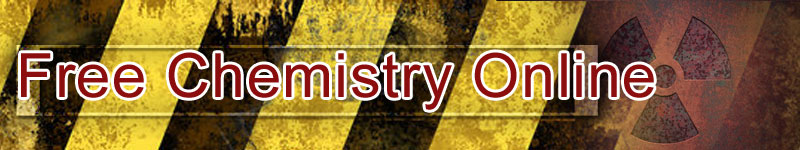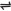## What is Salt?

A salt can be defined as a compound made up of positive metallic ions and negative ions derived from an acid.

### Types of Salts

There are different kinds of salts. These include:

1. Normal salt

The hydrogen ions of the acid are completely replaced by metallic ions . Examples are NaCl, CuSO4, KNO3, and CaCO3. Normal salts are electrically neutral.

2. Acid salt

The salt still has hydrogen atom(s) from an acid which can further be replaced by metallic ions. Examples include: NaHSO4, NaHCO3 and NaHS

3. Basic salt

The salt contains hydroxides together with metallic ions and negative ions from an acid. Examples are basic zinc chloride, ZnOHCl, basic magnesium chloride.

4. Double salt

Salt that ionizes to produce three different types of ions in solution, two of these are usually positively charged and the other negatively charged. Examples are ammonium iron(II) tetraoxosulphate(VI) hexahydrate, (NH4)2 Fe(SO4)2.6H2O; potash alum or aluminium potassium tetraoxosulphate(VI) dodecahydrate, KAl(SO4)2. 12H2O; and chrome alum or chromium(III) potassium tetraoxosulphate(VI) dodecahydrate, KCr(SO4)2. 12H2O.

5. Complex salt

The salt contains complex ions, i.e. ions consisting of a charged group of atoms. Examples are sodium tetrahydroxozincate(II)

 Na2Zn(OH)4(aq)2Na+(aq) + Zn(OH)2-4(aq)

potassium hexacyanoferrate(II)

 K4Fe(CN)6(aq)4K+(aq) + [Fe(CN)6]4-(aq)

### Hydrolysis of Salts

When salts dissolve in water they are hydrolyzed. The reaction between a salt and water to give either acidic or basic solution is known as hydrolysis.

Hydrolysis involves the split of water molecules into its ions, H+ and OH-. These ions then get attracted to the opposite ions of the salt. The degree of attraction determines which ion, i.e. H+ or OH- will be more in solution, thereby resulting in the solution being acidic (more H+ in solution) or basic (more OH- in solution) or neutral (equal conc. of H+ and OH- in solution).

The nature of the resultant solutions depends on the nature of the salts:

Salts formed from strong acids and strong bases (example, NaCl – HCl + NaOH)

These salts give neutral solutions (the H+ and OH- an attracted to the opposite ions of the salt in the same degree). Example, for the hydrolysis of NaCl, H+ and OH- ions are attracted at equal ease to Cl- and Na+ respectively to from HCl and NaOH.

Therefore, the solution contains equal concentration of H+ and OH- ions.

Salts formed from strong acids and weak bases (example, NH4Cl – HCl + NH3)

Solutions of these salts are acidic (i.e. conc. of H+ is more than that of OH-). The OH- ions are attracted more to the positive ions of the salts than the H+ ions are attracted to the negative ions.

Example, for the hydrolysis of NH4Cl, OH- ions are attracted more to NH4+ than H+ ions attracted to Cl- ions. Hence, there is more concentration of H+ ions in solution, which results in the solution being acidic.

Salts formed from weak acids and strong bases (e.g. NaHCO3 – NaOH + H2CO3 ; Na2CO3 – NaOH + H2CO3)

The solutions are basic (i.e. the concentration of OH- ions is more than that of H+ ions). This is because H+ ions are more attracted to the negative ions of the salt than OH- ions attracted to the positive ions of the salt.

Notice that the solution of NaHCO3 is basic, even though the salt is an acid salt. The name acid salt only means that there is or are still hydrogen atom(s) from an acid in the salt which can still be replaced by metallic ions. lt does not in all cases mean the solution of the salt is acidic.

lt will be acidic if the acid salt is formed from a strong acid, example, NaHSO4 (the hydrogen atom will be furnished in solution as the only positive ion).

Salts formed from weak acids and weak bases (e.g. (NH4)2CO3) The solutions of these salts are neutral (equal attraction between the positive ions of the salt and OH- ions of water; and the negative ions of salt and H+ ions of water. Hence equal concentration of H+ and OH- are in solution).

Note: hydrolysis also occurs in certain compounds which are not salts (examples are carbohydrates, fats and proteins). Therefore, hydrolysis can be defined generally as the reaction between the ions of water (i.e. H+ and OH-) and the opposite ions or poles of a substance formed with the loss of water molecules, to produce the reactants from which it was initially formed.

Example, when NaCl is hydrolysed, it produces HCl and NaOH - from which it was initially produced with the elimination of water molecules.